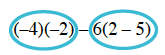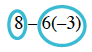### Home > CC3MN > Chapter 3 Unit 3 > Lesson CC3: 3.1.3 > Problem3-27

3-27.

1. $(−4)(−2)−6(2−5)$

Circle the terms.

Simplify within the circled terms.

Circle the terms again. Then simplify and repeat.$8−6(−3)$$26$

1. $23−(17−3·4)^2+6$

For parts (b), (c), and (d) follow the strategy outlined in part (a).

1. $14(2+3−2·2)\div(4^2−3^2)$

$2$

1. $12.7−18.5+15+6.3−1+28.5$